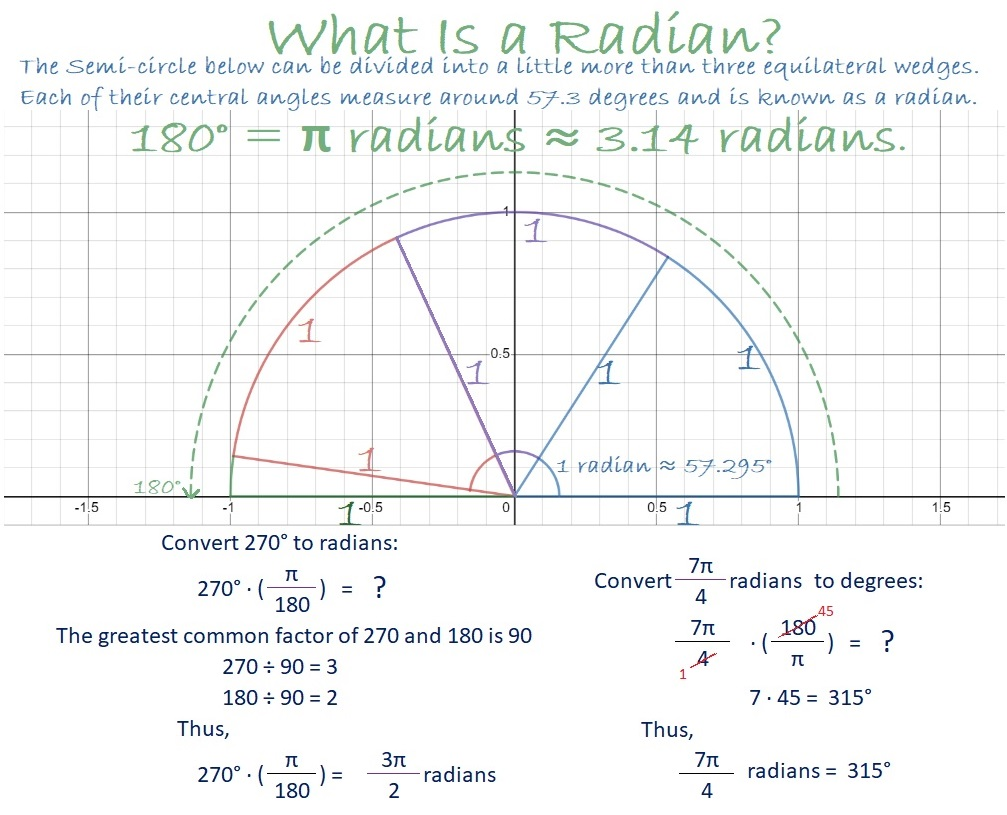# 1465 A Degrees to and from Radians Calculator

Contents

Elementary and middle school students measure their angles in degrees. When they get to high school, they find out that sometimes we prefer to measure angles in radians. Of course, they also need to convert degrees to radians and to convert radians into degrees.Since I have students who rely on calculators but don’t have access to one during this pandemic, I designed a calculator in Desmos to convert Degrees to and from Radians. The calculator doesn’t work like magic, still requires you to think about what you are doing, and should prevent some of the common errors in doing such conversions.

### Factors of 1465:

• 1465 is a composite number.
• Prime factorization: 1465 = 5 × 293
• 1465 has no exponents greater than 1 in its prime factorization, so √1465 cannot be simplified.
• The exponents in the prime factorization are 1, and 1. Adding one to each exponent and multiplying we get (1 + 1)(1 + 1) = 2 × 2 = 4. Therefore 1465 has exactly 4 factors.
• The factors of 1465 are outlined with their factor pair partners in the graphic below.### More Facts about the Number 1465:

1465 is the sum of two squares in two different ways:
36² + 13² = 1465
32² + 21² = 1465

1465 is also the hypotenuse of FOUR Pythagorean triples:
340-1425-1465 which is 5 times 68-285-293.
583-1344-1465 calculated from 36² – 13², 2(36)(13), 36² + 13².
879-1172-1465 which is (3-4-5) times 293.
936-1127-1465 calculated from 2(36)(13), 36² – 13², 36² + 13².

From OEIS.org, we learn this fun square number fact:
1465² = 2146225.

This site uses Akismet to reduce spam. Learn how your comment data is processed.